# Flow Rate Worksheet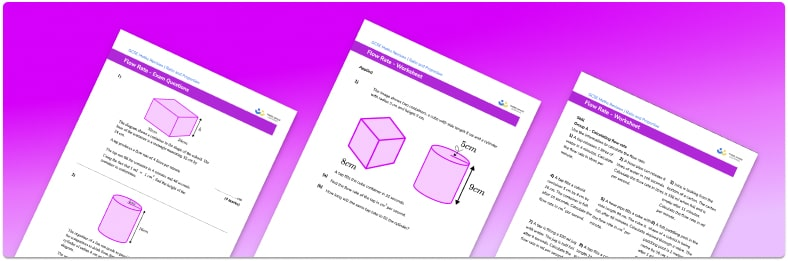• Section 1 of the flow rate worksheet contains 27 skills-based flow rate questions, in 3 groups to support differentiation
• Section 2 contains 4 applied flow rate questions with a mix of worded problems and deeper problem solving questions
• Section 3 contains 4 foundation and higher level GCSE exam style flow rate questions
• Answers and a mark scheme for all flow rate questions are provided
• Questions follow variation theory with plenty of opportunities for students to work independently at their own level
• All questions created by fully qualified expert secondary maths teachers

Suitable for high school GCSE maths revision for AQA, OCR and Edexcel exam boards

• This field is for validation purposes and should be left unchanged.

You can unsubscribe at any time (each email we send will contain an easy way to unsubscribe). To find out more about how we use your data, see our privacy policy.

### Flow rate at a glance

Flow rate measures how quickly liquid, such as water flows into or out of a container. The volumetric flow rate is measured in units of volume per time, for example litres per minute ( l/min ), gallons per minute ( gpm ) or millilitres per second ( ml/sec ).

Flow rate calculations can be done using the formula, \text{Flow rate} = \text{Volume}\div\text{Time} .

We can also calculate the volume or the time taken given the flow rate by substituting in the values we know and rearranging.

Consider oil passing through a 50cm circular pipe that has a radius of 2cm . The cross-sectional area of the pipe is 4\pi cm^2 and the volume when the pipe is full is 200\pi cm^3 . If the velocity of the oil through the pipe remains constant (the flow rate), we can determine the amount of oil passing through the pipe.

If the width of the cross-section of the pipe increases (the pipe diameter), more oil can pass through the pipe. The viscosity of a liquid affects the fluid flow rate (water is less viscous than custard and so water flows faster than custard). Hydrostatic pressure also affects flow rate because of the force of gravity.

We calculate flow rates in many real life contexts such as in medicine. The drop factor is the number of drops per millilitre of solution delivered by gravity. If the drip rate changes, the patient could receive too much of too little medicine over the allocated time period, which may affect their health.

Looking forward, students can then progress to additional rate of change worksheets and other ratio and proportion worksheets, for example a ratio worksheet or a simplifying and equivalent ratios worksheet.For more teaching and learning support on Ratio and Proportion our GCSE maths lessons provide step by step support for all GCSE maths concepts.

## Related worksheets

Sharing In A Ratio Worksheet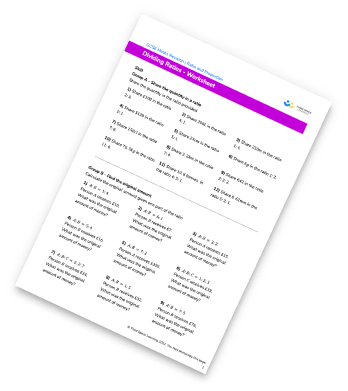Distance Time Graph Worksheet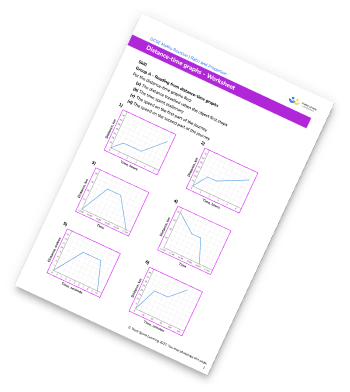Rate Of Change Worksheet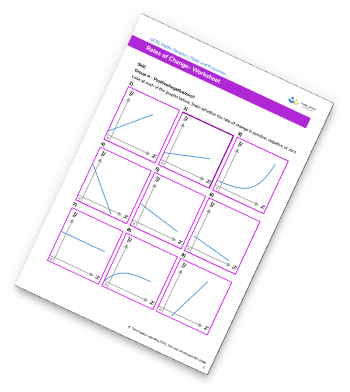Speed Time Graph Worksheet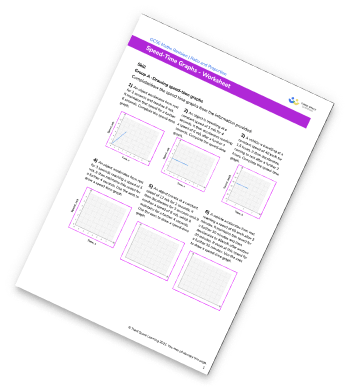## Do you have KS4 students who need more focused attention to succeed at GCSE?There will be students in your class who require individual attention to help them succeed in their maths GCSEs. In a class of 30, it’s not always easy to provide.

Help your students feel confident with exam-style questions and the strategies they’ll need to answer them correctly with our dedicated GCSE maths revision programme.

Lessons are selected to provide support where each student needs it most, and specially-trained GCSE maths tutors adapt the pitch and pace of each lesson. This ensures a personalised revision programme that raises grades and boosts confidence.

Find out more